# Inductive Logic

We can define any type of logic as a formal a priori system that is usually employed in reasoning. In general, if we feed in true propositions, and follow the rules of the particular system, the logic will crank out true conclusions.

We can define induction as a thought process that involves moving from particular observations of real world phenomena to general rules about all similar types of phenomena (a posteriori). We hold that these rules that we generate are probably, but not certainly, true, because such claims are not tautologies.

Inductive Logic therefore, is a formal system that can be distinguished from deductive logic in that the premises we feed into these arguments are not categories or definitions or equalities, but observations of the real world - the a posteriori world. Inductive logic therefore, is the reasoning we do every day, the probabilities that we deal with while making judgments about the world. We can think of it as learning from experience and applying our prior experiences to new, but similar, situations. We can also view it as one of the tools of science:"Quite frequently I encounter people who equate lack of certitude with giant inferential leaps. Science deals with probabilities, often quite high probabilities, but not certitudes. It is one of the strengths of the scientific method as it acknowledges a chance of error(while maintaining rigorous standards to establish provisional acceptance of propositions). It is a mistake to believe that a science consists in nothing but conclusively proved propositions, and it is unjust to demand that it should. It is a demand only made by those who feel a craving for authority in some form and a need to replace the religious catechism by something else, even if it be a scientific one. Science in its catechism has but few apodictic precepts (neccessary truths); it consists mainly of statements which it has developed to varying degrees of probability. The capacity to be content with these approximations to certainty and the ability to carry on constructive work despite the lack of final confirmation are actually a mark of the scientific habit of mind." -- Sigmund Freud

## History of Inductive Logic

It was the Greek Sophist philosopher Phyrro who first questioned the doctrine of the syllogism. He noted that the major premise of Aristotle's syllogisms take for granted precisely what they sought to 'prove', and that therefore, syllogisms could actually demonstrate nothing.

For example, consider the classic example of a syllogism:

Socrates is a man Man is a rational Animal Ergo, Socrates is a rational animal.

They key point of contention is this: If Socrates is not rational then it is not true that man is a rational animal in the first place.

Will Durant, from The History of Philosophy, futher explicates on this problem:

Aristotle would most likely have replied that where an individual is found to have a large number of qualities characteristic of the class (Socrates is a man) a strong presumption is established that the individual has the other qualities characteristic of that class (rationality). But a strong presumption remains a presumption. The syllogism, therefore, is not a mechanism for the discovery of truth so much as it is a method the clarification of exposition of thought - i.e. syllogisms allow us to demonstrate that, given an acceptance of the premises, the conclusion must follow. (Durant, 1926).

Therefore, from the time of the Greeks it grew increasingly clear that there was a need for a formalized method of working with the the probabilistic, a posteriori world. Inductive logic is basically a form of probability. While human beings have used intuitive forms of inductive reasoning all throughout history, probably theory was first formalized in 1654 by the mathematicians Pascal and Fermat - during their correspondence over the game of dice! In their attempts to understand the game, they created a set of frequencies - or possibilities that described the likelihood for particular rolls of the dice. In doing this, they accidentally set down the basics of probability theory.

It was only a short time later, in 1748, that someone noticed a problem in probability theory - that it included the presumption that the future would be just like the past, yet this assumption could not in of itself provide a sufficient condition for justifying induction, seeing as there is no valid logical connection between a collection of past experiences and what will be the case in the future. Hume's Inquiry Concerning Human Understanding" is noted, even today, for pointing out this problem - the "problem of induction". However, few realize that a solution to the problem appeared only a few years later: In 1763, Thomas Bayes's presented a theorem that unaware to him, could be used to provide a logical connection between the past and the future in order to account for induction. More recently, Kolmogorv (1933) axiomized probability theory, which means that he gave probability theory an axiomatic foundation. Induction, therefore, while a probabilistic enterprise, is founded on a deduced system:

## The three axioms of formalized probability theory

1. The probability of any proposition falls between 1 and 0.
2. The probability for a proposition with a possibility of 'certain' is 1
3. When there is no overlap, P(P or Q) = P(P) + P(Q)

and the definition of conditional probability:

P(P/Q) = P(P & Q)/P(Q)

If you accept these axioms, then you must accept Bayes Theorem. It follows logically from the axioms.

These are the key points to the history of induction as far as the formal origins and formal supports for induction. I will cover these points in more detail below. But first, let's look at the different types of inductive logic.

## Types of Inductive Logic

Let's do a brief review of some kinds of Inductive Logic

### Argument from analogy

This occurs when we compare two phenomena based on traits that they share. For example, we might hold that Object 'A' shares the traits w, x and y, with with object 'B,' therefore, object A might also share other qualities of object B.

### Statistical syllogism

This inductive logic is similar to the argument from analogy. The form of the logic follows: X% of "A" are "B", so the probability of "A' being "B" is X%

Example: 3% of smokers eventually contract lung cancer. John Doe is a smoker, therefore, he has a 3% chance of contracting lung cancer.

### Generalization from sample to population

The best example of this inductive logic would be a poll. Polls rely on random samples that are representative of a group by virtue of their random selection (i.e. the fact that every person had the same chance of being chosen for the sample).

For those further interested in induction,see my page on the scientific method: http://www.candleinthedark.com/scientific.html In addition, at the bottom of this section, I will also discuss John Stuart Mill's Method of Causality. The rest of this page will focus on the aformentioned "problem of induction" and take a deeper look both at the problem of induction, and some solutions for this problem.

### Simple induction

Simple induction proceeds from a premise about a sample group to a conclusion about another individual.

Proportion Q of the known instances of population P has attribute A.
Individual I is another member of P.
Therefore:
There is a probability corresponding to Q that I has A.

This is a combination of a generalization and a statistical syllogism, where the conclusion of the generalization is also the first premise of the statistical syllogism.

### Causal inference

A causal inference draws a conclusion about a causal connection based on the conditions of the occurrence of an effect. Premises about the correlation of two things can indicate a causal relationship between them, but additional factors must be confirmed to establish the exact form of the causal relationship.

### Prediction

A prediction draws a conclusion about a future individual from a past sample.

Proportion Q of observed members of group G have had attribute A.
Therefore:
There is a probability corresponding to Q that other members of group G will have attribute A when next observed.

## The Problem of Induction

You've probably heard about Hume's famous Problem of Induction. It may be worded thusly:

How do we know that the future will be like the past?

Or... more humorously:

How do we know that the future will continue to be as it always has been?!

The problem of induction points out that behind every claim lies a question of epistemology. In short, how do we justify the foundation for our inductive claims - Induction?

Consider the following example: we observe two billiard balls interact. From this, we observe that they appear to obey a physical law that could be presented in the formula: F=ma - Force = Mass X acceleration. From this observation, we then generate a general law of force. However, the problem then arises: how can we hold that this law will really apply to all similar situations in the future? How can we justify that this will always be the case?

If we argue that "we can know this, because the balls have always acted this way in the past" we are not really answering the question for the question asks how how we know that the balls will act this way in the future. Of course, we can then insist that the future will be just like the past, but this is the very question under consideration. We might next insist that there is a uniformity of nature that allows us to deduce our conclusion. But, how do we know that nature is uniform? Because in the past it always seemed so? Again, we are simply assuming what we seek to prove.

So, it turns out that this defense is circular... we assume what we seek to justify in the first place, that the past will be like the future. So this argument fails to provide a justification for induction.

### UON- A Non Solution to the Problem

As Howson & Urbach point out, assuming a uniformity of nature is a nonsolution, since it's a fairly empty assumption. For how is nature uniform? And what, really, are we talking about. What would really be needed are millions upon millions of uniformity assumptions for each item under discussion. We'd need one for the melting temperature of water, of iron, of nickel, etc, etc. For example "block of ice x will melt at 0 Celsius;" for these types of assumptions actually say something. Furthermore, the uniformity of nature assumptions fall prey to meta-uniformity issues - for how are we to know that nature will always be uniform? Well, we have to assume that too. And how do we know that the uniformity of nature is uniform? Ad infinitum. So, to "solve" the philosophical problem of justifying induction by uniformity of nature solutions doesn't really work.

Well then, if even logicians agree that the UON cannot justify induction, it would seem that the situation is hopeless. And it would be, if the claim that the UON cannot justify induction itself wasn't a [red herring].

The assumption of an UON does not address that actual problem of induction, because the actual problem relates to justifying moving from a set of observations to a general rule. Even if we accepted the UON as true, this assumption would not, in of itself, provide a framework for moving from observations to a general rule. To do this, we need a set of rules grounded in deductive logic.

## The Actual 'Problem of Induction'!

So, as a general rule, if you hear someone claim that the UON is used by 'logicians' to 'justify induction', you can be fairly certain that you're dealing with someone ignorant of the very basics of what logic is... As you can now see, the uniformity of nature is in fact a necessary condition for induction but it could never be a sufficient justification of inductive inference. The fact that the assumption of a uniformity of nature (UON) is of no help in justifying induction is not a concern to logicians in the first place, seeing as the actual problem of induction has nothing to do with the assumption of a uniformity of nature in the first place! The actual problem of induction is the claim that there is no valid logical connection between a collection of past experiences and what will be the case in the future. The classic white swans example serves: the fact that every swan you've seen in the past was white means simply that: every swan you've seen has been white. There is no logical "therefore" to bridge the connection "all the swans I've seen are white" to all swans are white or "the next swan I encounter will be white".

So, yes induction presupposes the uniformity of nature, but while this is a necessary condition for induction, the UN is not sufficient condition for justifying induction, nor is it considered to be a sufficient condition by modern logicians. So, any attempt to solve the problem by shoring up the 'uniformity of nature' will never work. When the next swan turns out to be black, it shows your statement "all swans are white" had no actual "knowledge" content. What you've done is presupposed nature to be uniform, but not in fact justified any particular inductive inference you may wish to make.

So, again, solving the 'problem' of induction is more than just trying to find a way out of the 'circle' of uniformity of nature/justifying induction. There is a problem that needs a solution. Interestingly, many critics seem to believe that the story ends here - that there simply is a problem, and that all solutions are merely circular. But this is untrue. There are responses to the problem.

Since it was Hume who first uncovered this problem, let's begin by looking at his response:

## Solutions to the Problem

### Induction is a Habit

Hume's answer was that we had little choice but to assume that the future will be like the past..... in other words, it was a habit born of necessity - we'd starve without it! And, given that there was nothing contradictory, nothing logically impossible or irrational to holding to a behavior through its utility, the utility of induction was seen to support induction a pragmatic basis.

It is important to remember that even without an epistemological foundation for induction, there is nothing illogical or irrational about assuming that induction works, nor is there in a purported lack of a epistemological foundation a rational grounds for holding that 'induction is untrustworthy'. The fact that we cannot be absolutely certain that the sun will rise tomorrow does not give me any justification in holding that it will not rise tomorrow! It merely tells us that we cannot be certain that it will rise tomorrow. Even if there were no justification for induction, this would not imply that we are without any knowledge that induction works.

But merely holding that an assumption is 'not irrational' is not a satisfying enough answer for many. People often prefer to move past pragmatism to a deeper, more philosophically satisfying answer. Hume himself stated: "As an agent I am satisfied but as a philosopher I am still curious." So let's continue our search for an answer to the problem.

### Probability Theory

As already mention above, Kolmogorv axiomized probability theory in 1933, giving probability theory (Induction) an axiomatic foundation. Induction, therefore, while a probabilistic enterprise, is founded on a deduced system.

Curiously however, the axiomatic foundations for inductive logic only tell us how a probability behaves, not what it is. So let's begin our examination by first defining what we actually mean by saying the word "probability".

#### Three Common Definitions of Probability

##### Classical

The classical definition describes probability as a set of possible occurrences where all possibilities are 'equally likely' - but a problem arises from this definition. For example, how do you define "possibility" in a univocal manner? Is an outcome 50/50 (either it happens or it does not) or is an outcome actually 1/10, 1/100? In many cases there are possible reasons for each choice. So let's look at another definition.

##### Frequentist

The 'frequency' is the probability for a given event, that is determined as you approach an infinite number of trials. For example, as with the central limit theorm, you could learn what a probability might be for the roll of a 7 on a pair of dice, after rolling them for a large number of trials. This is the most popular definition, including in science and medicine. This view is backed up by axiomatic deduced probability theory (based on infinite trials (like coin flips)) the law of large numbers. The frequency converges to the probability when we reach infinity. But there are problems here as well: does the limit actually exist? Do we ever really know a probability, since we can't do things infinitely? Also, this method gives us very counterintuitive interpretations. For example, consider a 95% confidence interval - often this is read to mean that 1 out of every 20 such studies is in error. In actuality, what this means is that if the experiment were repeated infinitely, you'd get the real mean 95% of the time. This is hardly what people think when they read a poll.

Finally, we can't apply this method to singular cases. 'One case probabilities' are "nonsense" to the frequentist. How do we work out the probability of the meteor that hit the earth to kill the dinosaurs?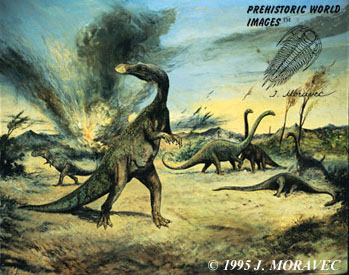We can't repeat this experiment infinitely! We can't repeat it once! We see the same problem with creationist arguments for our universe that attempt to assign a probability to the universe.

##### Subjective probability

Here, probability is held to be the degree of belief in an event, fact, or proposition. Look at the benefits of this model. 1) We can more carefully assign a probability to a given situation. 2) We can apply this to method 'one case events'. 3) This manner of defining probability gives us very natural and intuitive interpretations of events that fits with our use of the word "probably", circumventing the problems of frequentism. Most importantly, it allows us to rationally adjust our beliefs "inductively" by use of probability theory, which is a mathematically deduced theory, so we can latch on our beliefs onto a deductive axiomatic system. Here then, for many, is the solution to Hume's "problem" - induction is no longer merely "not irrational', but instead, can be seen as resting upon a firm deductive foundation.

## How Subjective Probability Works

How do you get a 'number' or probability, for subjective probability?

Let's use the concept of wagering.... What would you consider to be a fair bet for a particular outcome? Is X more probable then getting Y heads in a row in your view? In brief, this is how the method works.

Subjective probability and frequency are linked by Ian Hacking's Frequency Principle. Subjective probability is justified by a reductio argument: if your subjective probabilities don't match the frequency, and you know nothing else, you have no grounds for your belief.

A question may arise: How can we reason anything if probability's subjective? Well, it is true that you can just choose any starting ground you desire, however, your choice must follow laws of probability, or else you're susceptible to Dutch Book Arguments In gambling a Dutch book is a set of odds and bets which guarantees a profit, regardless of the outcome of the gamble. It is associated with probabilities implied by the odds not being coherent. So, what this means is that if your degrees of belief don't follow the laws of probability, you are being inconsistent and incoherent. You can choose to believe what you want, but at the risk of being incoherent. The beauty of this method is that a starting point is not necessarily very important: given differing starting probabilities, based on different subjective evaluations, two very different people who are shown enough of the same evidence will have their probabilities converge to the same value (The Law of Large Numbers).

Being a subjectivist who wants to use probability as a basis of induction leads us to focus on a certain way of doing things using, Bayes' Theorem.

## Bayes' Theorem

The simplest form of Bayes' Theorem: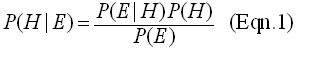where:

H is is the hypothesis. This is a falsifiable claim you have about some phenomena in the real world

E is the evidence This it the reason or justification you have for holding to the hypothesis. In rhetorical terms, it is your grounds for your claim.

P(E|H) is called the likelihood : it is also the probability of E given H. In other words, it is the probability that the evidence would occur if the hypothesis were true. If someone's claim necessitates that evidence abound, and evidence is scant, this alone may sink a hypothesis.

P(H) is called the prior, or prior probability of H. It is the probability of the hypothesis being true without taking additional evidence into consideration. In other words, it is an unconditional probability. When I call something, "the prior" without qualification, I mean this probability.

P(E) is called the prior probability of the evidence E. It is the probability of E occurring regardless of H being true. This probability can be broken down further into the partition , as explained below.

The denominator of Equation 1 (P(E)) can be broken down as: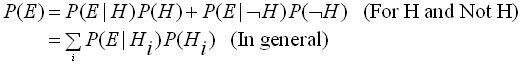where H is the compliment of H, AKA not-H, and S is the sum over all independent hypotheses. This is sometimes called [i]the partition[/i]. The top form is used when one is only considering whether a hypothesis H is true or false. The bottom form is more general, and holds for several independent hypotheses.

Plugging these into Equation 1 yields either: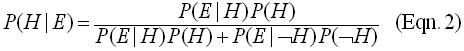which is useful when considering one hypothesis, being either true or false - this denominator of the right side of the equation multiplies the probability of the hypothesis being true against the probability of the hypothesis being false.

or it yields: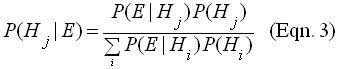This, in a nutshell, is a possible foundation for Inductive logic.

Notice that this system allows us to rule in and rule out a claim. Bayesian logic implies that for two competing hypotheses, H and not-H, absence of evidence for H would in fact be evidence for not-H.

## Notes of Bayes

Rev. Bayes may have (but not definitely) disagreed with "subjective probability". He derived his equation in order to answer a weird problem, which is briefly as follows: you have a pool table of a known size. You draw a line across it parallel to one of the edges (I forget if it's the long or short edge). But you don't know where along the pool table the line's drawn. Now, you place a billiard ball on the table "at random" (equal probabilities of it being anywhere on the table), and you get a yes or no answer to the following question each time you do it: "is the ball to the left of the line?". Repeat this process a few times. With this problem, Bayes derived his equation and used it to find the probability that the line is drawn at distance X from one side of the table: i.e. the probability that the line is X away from one side of the table.

So, whiles Bayes' theorem can be called upon to solve the problem of induction, Bayes wasn't really concerned with induction. He laid the mathematical foundations, however, for it to be "solved" (many people still today say that Bayesianism isn't really a solution, but a circumvention, of the problem of induction - a very technical point, however. And some object to Bayesianism altogether). The mathematician Pierre Laplace was the one who took up subjective probability and ran with it: he calculated the probability of the mass of a planet with it, and even calculated the probability that the sun would in fact rise tomorrow. There were, however, fatal flaws in his argument which led subjective probability to be all but abandoned. The frequentists took up the ball, and ran with it, until the mathematician Bruno De Finetti picked up Laplace's torch, leading to "Bayesianism" almost as we know it today.

## Conclusion

Gregroy Lopez believes that both classical and Bayesian statistics answer the problem of induction, as they are both founded on a priori deductive systems. Thus, he ultimately believes that the problem of induction is only a problem if one wishes to find certainty in a belief, and nothing more. It completely discounts degrees of belief.

Degrees of belief is most directly addressed by the Bayesian view. However, the frequentist interpretation still has some power against the problem of induction in my view as well.

In short - no matter how one ultimately slices it, the mathematics of probability and statistics ultimately does away with the problem of induction - Bayesian or not.

At the same time, it is an error to suppose that a lack of an adequate justification for induction would render induction worthless, or require that we hold to induction without any reason at all. This is a non sequitur. Christian Presuppositionalists mistakenly hold that a failure to provide an adequate justification for induction leaves us without any grounds to rely on induction other than 'faith', but this is nonsense: The fact one cannot prove something to be correct doesn't imply that one cannot know that the system is correct. A child is unable to prove his name, does this mean he does not know it? Knowledge and proof are two different philosophical concepts. The Problem of Induction relates to philosophical justification.

In the end, no one outside of a Christian Presuppositionalist confuses the problems of induction as a grounds for great concern. Usually when people talk about how induction is "flawed," they mean that it's not truth-preserving like deduction. You don't get certainty conclusions from an inductive argument. But if you accept that induction is necessarily tentative; if you don't look for certainty, and you know about modern probability and statistics, the problem of induction is not a problem at all. The whole (deductively-created) theory of probability and statistics is dedicated to telling us something about "populations" from "samples." It's made for induction.

See Also: John Stuart Mill and his Methods of Induction

## References

Copi, I. M, Cohen, C., (2001), "Introduction to Logic", 11th Edition.

Durant, W. (1926) The Story of Philosophy.

Lopez, G. "Inductive Logic" (2006) A live presentation on the Infidelguy.com internet radio show, original broadcast: Feb 16th, 2006.